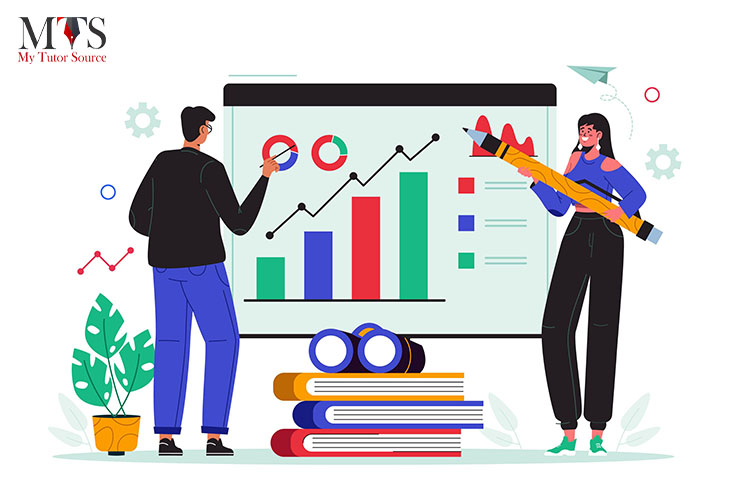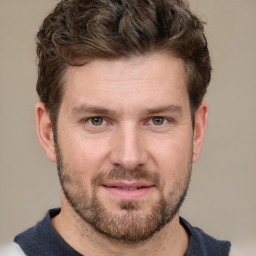# How To Learn Statistics From Scratch?

• Published On:Before diving into the tips and tricks of learning statistics, we must know what it is and why we should learn it. Statistics is the science of collecting and analyzing data for inferring proportions in a whole from those in a representative sample. In simpler words, statistics is using data to come up with conclusions.

As for the question, "why should we learn statistics?". There are two main reasons why studying statistics is essential in modern-day society. Statistics are guides for learning from data and cruising through common issues that can lead you to wrong conclusions. It is also crucial that you can assess the quality of analysis that others present to you.

You may have come across statistics somewhere in school or at university. You will have a minimal understanding of statistics unless you are pursuing a degree in statistics.

When people think about statistics, they often imagine a boring course they must study to complete their education. Because most often, people have no idea of what they are talking about and have drawn their conclusions from introductory statistics courses, which seem dull. Statistics has a lot of exciting mathematics if you manage to look in the right places.

I will tell you what steps you should take if you have zero knowledge of statistics but want to learn. Here are some tips to make your learning more efficient and fruitful.

You are mistaken if you can grind and wrap up the entirety of statistics in a single sitting. Statistics being a branch of mathematics, uses a ton of mathematical concepts. But you do not have to be an expert in mathematics to understand statistics.

Informative Read: How To Put Learning Into Execution?

You must be comfortable with math topics such as linear algebra and calculus. Knowing basic set theory will also be an advantage. For linear algebra, the textbook "Linear algebra and its applications" by Gilbert Strang. For calculus (including multivariable calculus), I recommend "Calculus: early transcendentals" by James Stewart.

## Get A Grip On The Basics Of Statistics And What It Can Do

We often think of conclusions from statistical analysis as absolute truths. Statistics do not prove anything but instead point toward conclusions.

A typical example of this is if both events A and B happen, that is, they are correlated. It does not mean that A causes B or vice-versa. You must know what techniques are appropriate for a specific analysis. To be able to do this, you need to be aware of the advantages and limitations of using statistics.

The first step is getting a feel of descriptive statistics and what measures we can use to quantify data. There are three main types of descriptive statistics: distribution, central tendency, and variability.

The distribution is concerned with the frequency of each value. The central tendency involves the averages of the values. The variability concerns how spread out the values are.

You also need to be aware of how to perform calculations with these concepts. These are usually done in spreadsheet software. A great book to get you started is "The Art of Statistics: How to Learn from Data" by David Spiegelhalter.

## Become Acquainted With Probabilistic Models And Inferential Statistics

Once you understand basic descriptive statistics, you can plunge into probabilistic models. In schools, we are taught probability independently of statistics. The truth is that probability forms a significant part of statistical analysis.

When we hear the word probability, we think of combinatorial probability, "if I draw three cards at random from a deck of playing cards, what is the probability that I get three hearts in a row?

Problems like these give you a basic understanding of how probability works, but they are pretty useless in statistics.

The key things you need to learn about are probability distributions. In hypothesis testing, we need to test whether a particular hypothesis for the relation between observations has enough evidence to support it. This evidence can be found by applying a specific probability distribution.

The common probability distributions are Bernoulli, Uniform, Binomial, Normal, Poisson, and Exponential Distribution. "A First Course in Probability" by Sheldon Ross covers everything you will need to know about probability. Another great resource can be found on this website. https://www.probabilitycourse.com

## Start Learning Predictive Modeling, Linear, And Logistic Regression

Predictive modeling, also called predictive analytics, is used to make future predictions based on past information from data. Datasets are studied for patterns and trends that can be used to create a model of potential future outcomes.

Linear regression is the simplest and most used form of regression analysis. A linear equation is a model for the relationship between two variables. One independent variable is referred to as the explanatory variable. The other is the dependent variable, and its value depends on the first. In logistic regression, one of the variables does not have a numerical value.

Regression analysis uses a lot of linear algebra and calculus to derive expressions for the lines of best fit to a dataset. A book to start with is "Regression Analysis: A Practical Introduction" by Jeremy Arkes. For a more theoretical introduction, "Regression Analysis: Theory, Methods, and Applications" would work.

"Chi-Squared data analysis and model testing for beginners" is also a great book.

## What Are The Next Steps?

Statistics is a valuable asset that can benefit individuals in many areas of their lives. Once you get past the initial stages of learning statistics, you will then have a grip on what statistics is.

The next step would be to delve into Multivariate statistics after completing the basics, with concepts like principal components analysis (PCA). Another thing that will be of great use to many is the Monte Carlo methods, which allows you to simulate and predict the outcome of repeated trials.

There are countless things to learn in the world of statistics. The following steps depend on your needs/interests. The methods at this stage are more specialized and depend on your area of interest. Once you have mastered the basics, everything should be a lot easier.

## Conclusion

This is the perfect way to start learning about statistics. Start with mathematical concepts, then descriptive statistics, followed by inferential statistics, and then predictive modeling. I hope this guide aids you in achieving your goal of learning statistics.

### Find Top Tutors in Your AreaAustin has 10+ years of experience in teaching. He has researched on thousands of students-related topics, issues, and concerns. You will often find him writing about the common concerns of students, their nutrition, and what is beneficial for their academics and health both.